# Plotting polar curves in Python

A point in polar co-ordinates is represented as (r, theta). Here, r is its distance from the origin and theta is the angle at which r has to be measured from origin. Any mathematical function in the Cartesian coordinate system can also be plotted using the polar coordinates.

### Modules required

• Matplotlib : Matplotlib is a comprehensive Python library for creating static and interactive plots and visualisations. To install this module type the below command in the terminal.
pip install matplotlib

• Numpy : Numpy is the core library for array computing in Python. To install this module type the below command in the terminal.
pip install numpy

• math : math is a built-in module used for performing various mathematical tasks.

The matplotlib.pyplot module contains a function polar(), which can be used for plotting curves in polar coordinates.

Syntax : matplotlib.pyplot.polar(theta, r, **kwargs)

Parameters :

• theta – angle
• r – distance

Approach :

In each of the examples below,

• A list of radian values is created. These values cover the domain of the respective function.
• For each radian value, theta, a corresponding value of r is calculated according to a specific formula for each curve.

1. Circle : A circle is a shape consisting of all points in a plane that are a given distance(radius) from a given point, the centre. Hence, r is a constant value equal to the radius.

Example :

## Python3

 import numpy as np  import matplotlib.pyplot as plt        # setting the axes projection as polar  plt.axes(projection = 'polar')     # setting the radius  r = 2    # creating an array containing the  # radian values  rads = np.arange(0, (2 * np.pi), 0.01)     # plotting the circle  for rad in rads:      plt.polar(rad, r, 'g.')     # display the Polar plot  plt.show()

Output :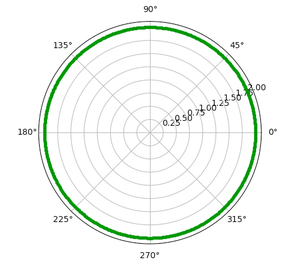2. Ellipse : An ellipse is the locus of a point moving in a plane such that the sum of its distances from two other points (the foci) is constant. Here, r is defined as :Where,

• a = length of semi major axis
• b = length of semi minor axis

Example :

## Python3

 import numpy as np  import matplotlib.pyplot as plt  import math        # setting the axes  # projection as polar  plt.axes(projection = 'polar')     # setting the values of  # semi-major and  # semi-minor axes  a = 4 b = 3    # creating an array  # containing the radian values  rads = np.arange(0, (2 * np.pi), 0.01)     # plotting the ellipse  for rad in rads:      r = (a*b)/math.sqrt((a*np.sin(rad))**2 + (b*np.cos(rad))**2)      plt.polar(rad, r, 'g.')     # display the polar plot  plt.show()

Output :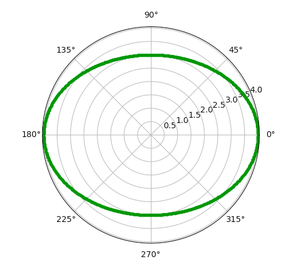3. Cardioid : A cardioid is the locus of a point on the circumference of a circle as it rolls around another identical circle. Here, r is defined as :Where, a = length of axis of cardioid

Example  :

## Python3

 import numpy as np  import matplotlib.pyplot as plt  import math        # setting the axes  # projection as polar  plt.axes(projection = 'polar')     # setting the length of   # axis of cardioid  a=4    # creating an array  # containing the radian values  rads = np.arange(0, (2 * np.pi), 0.01)      # plotting the cardioid  for rad in rads:      r = a + (a*np.cos(rad))       plt.polar(rad,r,'g.')      # display the polar plot  plt.show()

Output :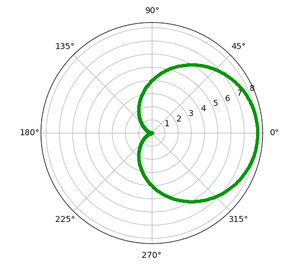4. Archimedean spiral : An Archimedean spiral is the locus of a point moving uniformly on a straight line, which itself is turning uniformly about one of its end points. Here, r is defined as :Example:

## Python3

 import numpy as np  import matplotlib.pyplot as plt        # setting the axes  # projection as polar  plt.axes(projection = 'polar')     # creating an array  # containing the radian values  rads = np.arange(0, 2 * np.pi, 0.001)      # plotting the spiral  for rad in rads:      r = rad      plt.polar(rad, r, 'g.')         # display the polar plot  plt.show()

Output :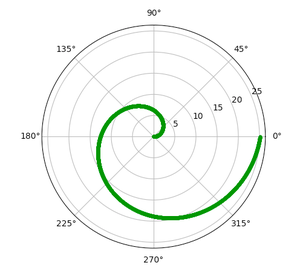5. Rhodonea : A Rhodonea or Rose curve is a rose-shaped sinusoid plotted in polar coordinates. Here, r is defined as :Where,

• a = length of petals
• n = number of petals

Example:

## Python3

 import numpy as np  import matplotlib.pyplot as plt        # setting the axes  # projection as polar  plt.axes(projection='polar')     # setting the length  # and number of petals  a = 1 n = 6    # creating an array  # containing the radian values  rads = np.arange(0, 2 * np.pi, 0.001)      # plotting the rose  for rad in rads:      r = a * np.cos(n*rad)      plt.polar(rad, r, 'g.')      # display the polar plot  plt.show()

Output :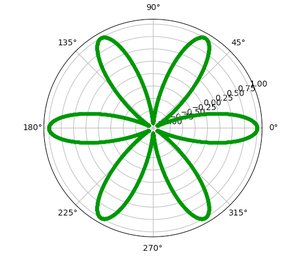My Personal Notes arrow_drop_upCheck out this Author's contributed articles.

If you like GeeksforGeeks and would like to contribute, you can also write an article using contribute.geeksforgeeks.org or mail your article to contribute@geeksforgeeks.org. See your article appearing on the GeeksforGeeks main page and help other Geeks.

Please Improve this article if you find anything incorrect by clicking on the "Improve Article" button below.

Article Tags :

Be the First to upvote.

Please write to us at contribute@geeksforgeeks.org to report any issue with the above content.